## Example Questions

1 3 Next →

### Example Question #674 : Algebra

Choose the answer which best simplifies the following expression: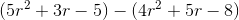Possible Answers: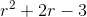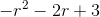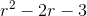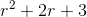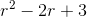Correct answer:Explanation:

To simplify, remove parentheses and combine like terms: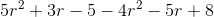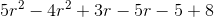### Example Question #675 : Algebra

Choose the answer which best simplifies the following expression: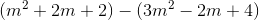Possible Answers: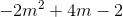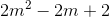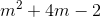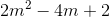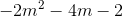Correct answer:Explanation:

To simplify, remove parentheses and combine like terms: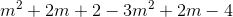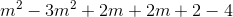### Example Question #1 : How To Divide Trinomials

Simplify the expression: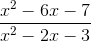Possible Answers: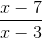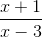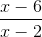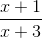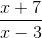Correct answer:Explanation: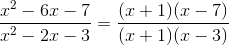Once simplified, (x+1) appears on both the numerator and denominator, meaning we can cancel out both of them.

Which gives us:### Example Question #2 : How To Divide Trinomials

Divide: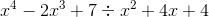Possible Answers: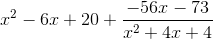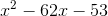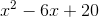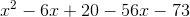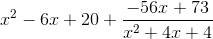Correct answer:Explanation:can be divided using long division.

The set up would look very similar to the division of real numbers, such as when we want to divide 10 by 2 and the answer is 5.

The first step after setting up the "division house" is to see what the first term in the outer trinomial needs to multiplied by to match the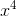in the house. In this case, it'swill be multiplied across the other two terms in the outer trinomial and the product will be subtracted from the expression inside the division house. The following steps will take place in the same way.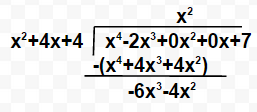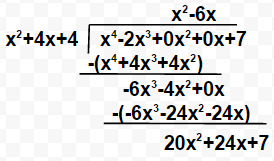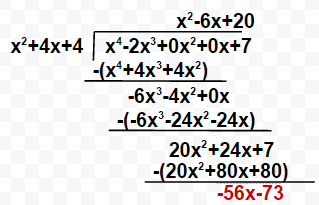While we could continue to divide, it would require the use of fraction exponents that would make the answer more complicated. Therefore, the term in red will be the remainder. Because this remainder is still subject to be being divided by the trinomial outside of the division house, we will make the remainder part of the final answer by writing it in fraction form: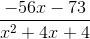Therefore the final answer is1 3 Next →

### All ACT Math Resources